# Understanding Logistic Regression

If you are interested in running the code I used for this analysis, please check out my GitHub.
Logistic regression is one of the foundational tools for making classifications. And as a future data scientist, I expect to be doing a lot of classification. So I figured I better understand how logistic regression functions at a deeper level (beyond just “from sklearn.linear_model import LogisticRegression”).

The following example walks through a very basic logistic regression from start to finish so that I (and hopefully you, the reader) can build more intuition on how it works.

Let’s say I wanted to examine the relationship between my basketball shooting accuracy and the distance that I shoot from. More specifically, I want a model that takes in “distance from the basket” in feet and spits out the probability that I will make the shot.

First I need some data. So I went out and shot a basketball from various distances while recording each result (1 for a make, 0 for a miss). The result looks like this when plotted on a scatter plot: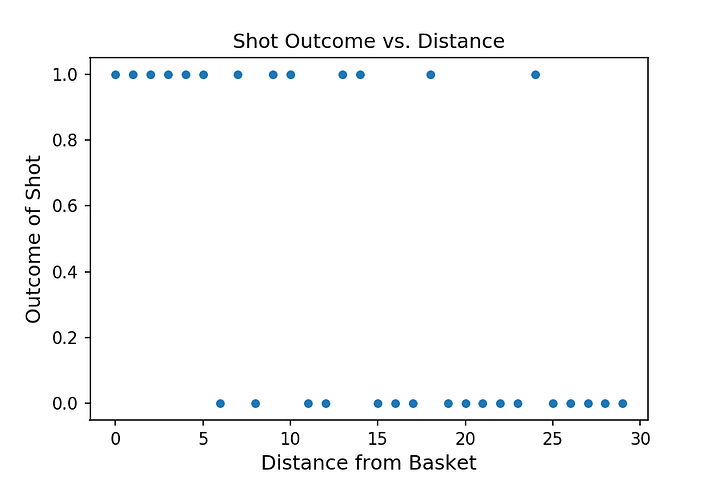Generally, the further I get from the basket, the less accurately I shoot. So we can already see the rough outlines of our model: when given a small distance, it should predict a high probability and when given a large distance it should predict a low probability.

At a high level, logistic regression works a lot like good old linear regression. So let’s start with the familiar linear regression equation:

Y = B0 + B1*X

In linear regression, the output Y is in the same units as the target variable (the thing you are trying to predict). However, in logistic regression the output Y is in log odds. Now unless you spend a lot of time sports betting or in casinos, you are probably not very familiar with odds. Odds is just another way of expressing the probability of an event, P(Event).

Odds = P(Event) / [1-P(Event)]

Continuing our basketball theme, let’s say I shot 100 free throws and made 70. Based on this sample, my probability of making a free throw is 70%. My odds of making a free throw can be calculated as:

Odds = 0.70 / (1–0.70) = 2.333

So if they basically tell us the same thing, why bother? Probabilities are bounded between 0 and 1, which becomes a problem in regression analysis. Odds as you can see below range from 0 to infinity.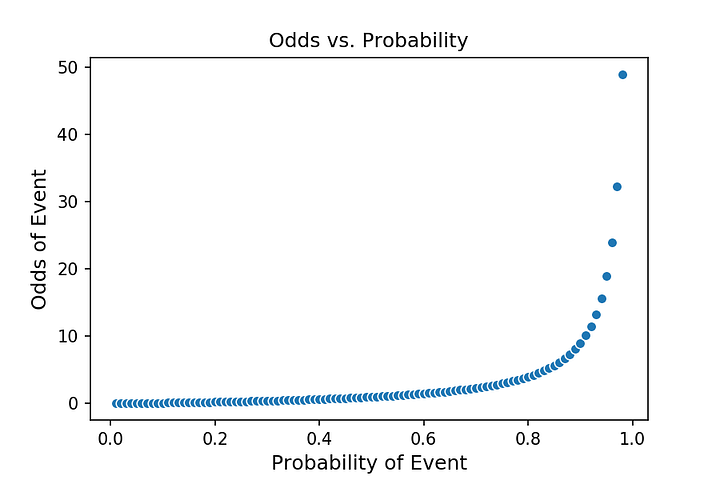And if we take the natural log of the odds, then we get log odds which are unbounded (ranges from negative to positive infinity) and roughly linear across most probabilities! Since we can estimate the log odds via logistic regression, we can estimate probability as well because log odds are just probability stated another way.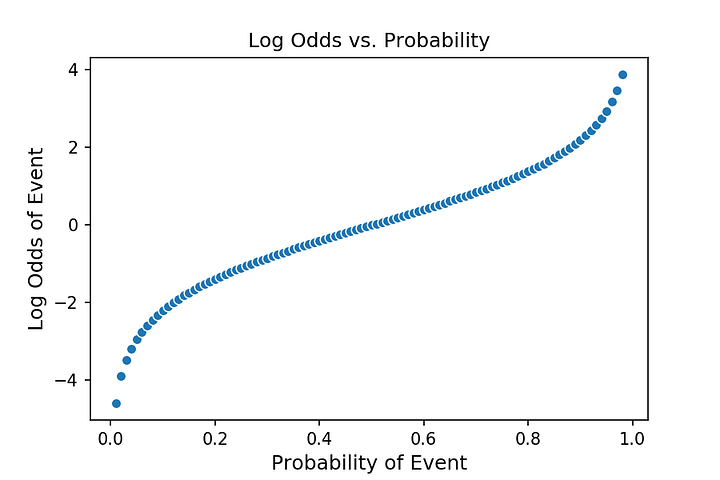Notice that the middle section of the plot is linear

We can write our logistic regression equation:

where Z = log(odds_of_making_shot)

And to get probability from Z, which is in log odds, we apply the sigmoid function. Applying the sigmoid function is a fancy way of describing the following transformation:

Probability of making shot = 1 / [1 + e^(-Z)]

Now that we understand how we can go from a linear estimate of log odds to a probability, let’s examine how the coefficients B0 and B1 are actually estimated in the logistic regression equation that we use to calculate Z. There is some math that goes on behind the scenes here, but I will do my best to explain it in plain English so that both you (and I) can gain an intuitive understanding of this model.

# The Cost Function

Like most statistical models, logistic regression seeks to minimize a cost function. So let’s first start by thinking about what a cost function is. A cost function tries to measure how wrong you are. So if my prediction was right then there should be no cost, if I am just a tiny bit wrong there should be a small cost, and if I am massively wrong there should be a high cost. This is easy to visualize in the linear regression world where we have a continuous target variable (and we can simply square the difference between the actual outcome and our prediction to compute the contribution to cost of each prediction). But here we are dealing with a target variable that contains only 0s and 1s. Don’t despair, we can do something very similar.

In my basketball example, I made my first shot from right underneath the basket — that is [Shot Outcome = 1 | Distance from Basket =0]. Yay, I don’t completely suck at basketball. How can we translate this into a cost?
• First my model needs to spit out a probability. Let’s say it estimates 0.95, which means it expects me to hit 95% of my shots from 0 feet.
• In the actual data, I took only one shot from 0 feet and made it so my actual (sampled) accuracy from 0 feet is 100%. Take that stupid model!
• So the model was wrong because the answer according to our data was 100% but it predicted 95%. But it was only slightly wrong so we want to penalize it only a little bit. The penalty in this case is 0.0513 (see calculation below). Notice how close it is to just taking the difference of the actual probability and the prediction. Also, I want to emphasize that this error is different from classification error. Assuming the default cutoff of 50%, the model would have correctly predicted a 1 (since its prediction of 95% > 50%). But the model was not 100% sure that I would make it and so we penalize it just a little for its uncertainty.

-log(0.95) = 0.0513
• Now let’s pretend that we built a crappy model and it spits out a probability of 0.05. In this case we are massively wrong and our cost would be:

-log(0.05) = 2.996
• This cost is a lot higher. The model was pretty sure that I would miss and it was wrong so we want to strongly penalize it; we are able to do so thanks to taking the natural log.

The plots below show how the cost relates to our prediction (the first plot depicts how cost changes relative to our prediction when the Actual Outcome =1 and the second plot shows the same but when the Actual Outcome = 0).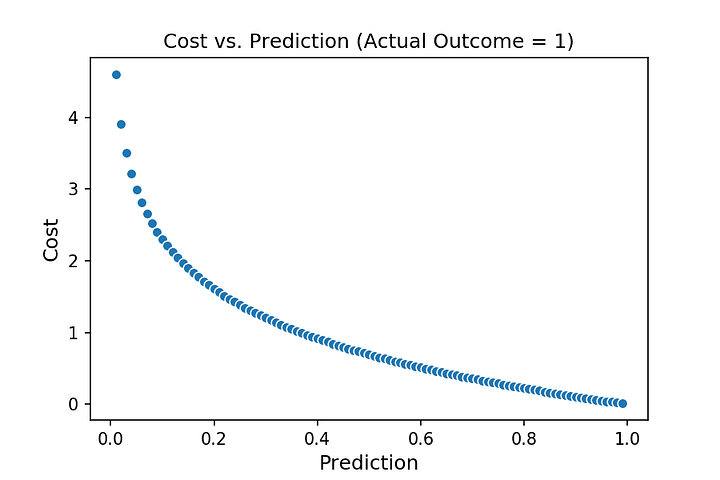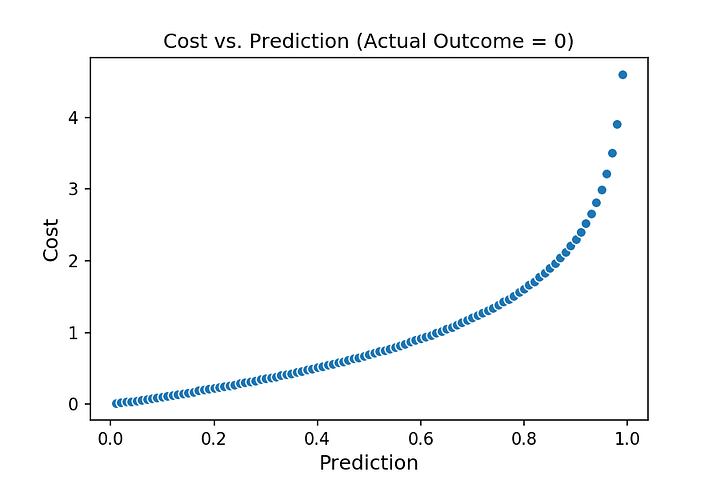So for a given observation, we can compute the cost as:
• If Actual Outcome = 1, then Cost = -log(pred_prob)
• Else if Actual Outcome = 0, then Cost = -log(1-pred_prob)
• Where pred_prob is the predicted probability that pops out of our model.

And for our entire data set we can compute the total cost by:
1. Computing the individual cost of each observation using the procedure above.
2. Summing up all the individual costs to get the total cost.

This total cost is the number we want to minimize, and we can do so with a gradient descent optimization. In other words we can run an optimization to find the values of B0 and B1 that minimize total cost. And once we have that figured out, we have our model. Exciting!

# Tying it All Together

To sum up, first we use optimization to search for the values of B0 and B1 that minimize our cost function. This gives us our model:

Z = B0 + B1*X
Where B0 = 2.5 and B1 = -0.2 (identified via optimization)

We can take a look at our slope coefficient, B1, which measure the impact that distance has on my shooting accuracy. We estimated B1 to be -0.2. This means that for every 1 foot increase in distance, the log odds of me making the shot decreases by 0.2. B0, the y-intercept, has a value of 2.5. This is the model’s log odds prediction when I shoot from 0 feet (right next to the basket). Running that through the sigmoid function gives us a predicted probability of 92.4%. In the following plot, the green dots depict Z, our predicted log odds.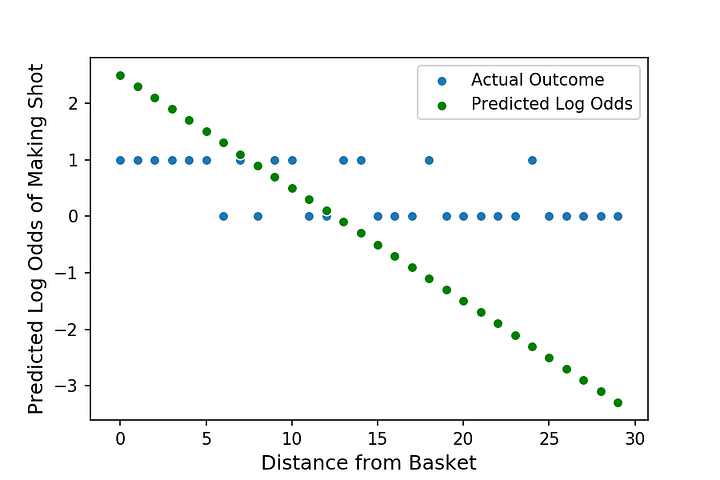Almost there!

We are almost done! Since Z is in log odds, we need to use the sigmoid function to convert it into probabilities:

Probability of Making Shot = 1 / [1 + e^(-Z)]

Probability of Making Shot,
the ultimate output that we are after is depicted by the orange dots in the following plot. Notice the curvature. This means that the relationship between my feature (distance) and my target is not linear. In probability space (unlike with log odds or with linear regression) we cannot say that there is a constant relationship between the distance I shoot from and my probability of making the shot. Rather, the impact of distance on probability (the slope of the line that connects the orange dots) is itself a function of how far I am currently standing from the basket.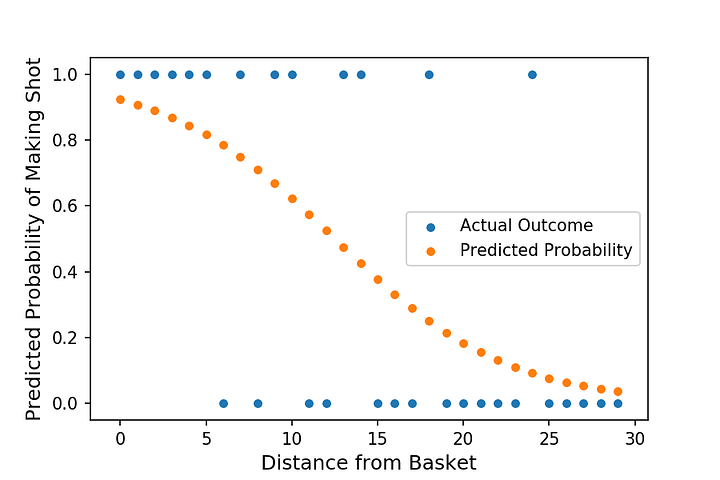Nice! We have our probabilities now

Hope this helps you understand logistic regression better (writing it definitely helped me).

More by me on Data Science Topics:

“Understanding Logistic Regression”
– Tony Yiu

### Related Stories

Nov 25, 2021

#### 5 Tips To Ace Your Job Interview For A Data Scientist Opening

5 Tips To Ace Your Job Interview For A Data Scientist Opening.PNG 795.94 KBImage SourceAspiring data scientists have a bright future ahead of them....By Daniel Morales
Nov 12, 2021

#### When to Avoid Deep LearningBy Matt Przybyla
Oct 16, 2021

#### 6 Advanced Statistical Concepts in Data Science

The article contains some of the most commonly used advanced statistical concepts along with their Python implementation.In my previous articles Be...By Nagesh Singh Chauhan